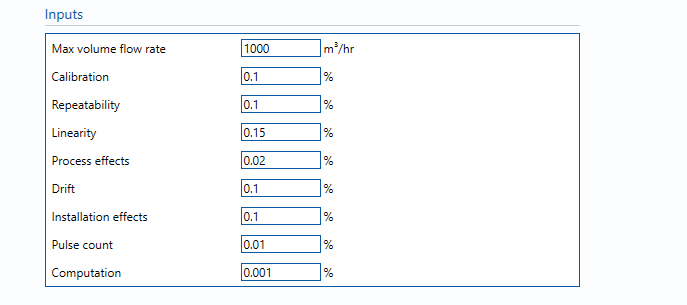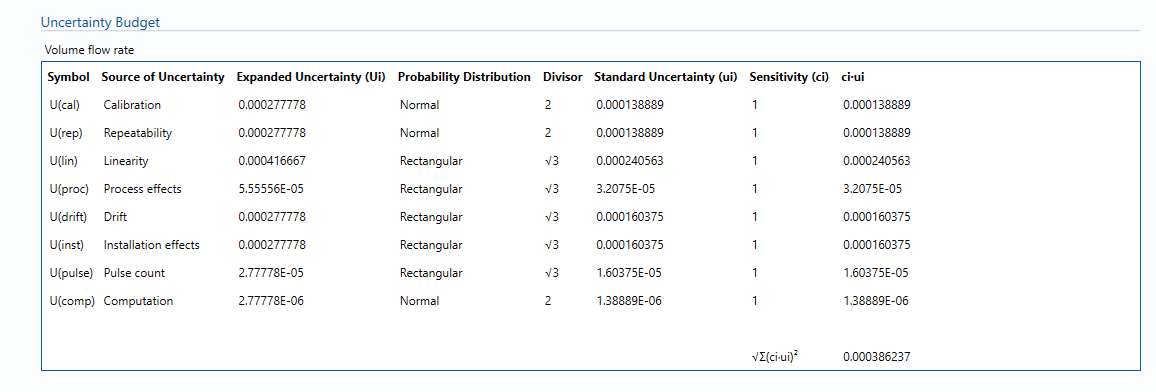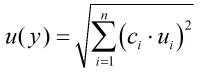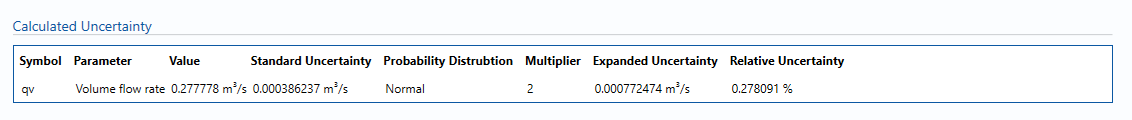# B405 – Turbine Meter Liquid Flow Rate

## Description

This block calculates the uncertainty in liquid flow rate through a turbine meter.

## InputsMaximum volume flow rate – Maximum turbine meter flow rate. Allows uncertainties, such as linearity, to be calculated as a function of maximum flow rate.

Calibration – Uncertainty in calibration reference device. Taken from calibration report and increased if deemed appropriate to consider T/P corrections etc.

Note: If not available assume 0.1%.

Repeatability – Uncertainty due to the meter short term repeatability, longer term reproducibility and hysteresis.

Note: Obtained from calibration data if available, otherwise assume 0.05%.

Process effects – Estimated uncertainty due to changes in process parameters between calibration and operation, such as temperature, pressure, density and, most significantly, viscosity.

Note: If not available assume 0.1%.

Drift – Maximum likely drift in meter factor between calibrations.

Note: Can only get this from repeat calibration data. If not available assume 0.1%.

Installation effects – Uncertainty due to non-ideal flow profile caused by upstream bends, valves, etc or general differences between lab and field piping configuration.

Note: Very difficult to estimate without test data from a replica installation (in a lab) or from CFD predictions. In the absence of either an assumption must be made, perhaps 0.1% but could be smaller/larger.

Pulse count – Uncertainty due to computational errors in number of pulses collected.

Note: Negligible term included for completeness, assume 0.01%.

Computation – Uncertainty due to computational errors in flow computer.

Note: Negligible term included for completeness, assume 0.001%.

## Calculations

The volume flow rate is taken from the Input/Output tab whilst the standard volume & mass flow rates are calculated from the volume flow rate using the VCF and line density respectively.

The VCF and line density values and their associated uncertainties are taken from the block B016 – Oil VCF.

The units selected for parameters on the Input/Output tab will be applied globally throughout the uncertainty module in use. They will be applied to values used in the uncertainty budget. Inputs that are local to this block can have their units altered within the block.

### Volume flow rate

The uncertainty calculation for the volume flow rate is detailed in the uncertainty budget table giving a break down of how the overall uncertainty is calculated.The values input into the uncertainty budget are taken from the uncertainty components calculated in this block from the calculation inputs. These values are taken in as the expanded uncertainties and are divided by a coverage factor to gain the standard uncertainty.

The coverage factor is determined by the probability distribution that best suits that uncertainty component. The standard uncertainty is then multiplied by the sensitivity value then squared. This is done for each component that contributes to the overall uncertainty in volume flow rate. The Standard Uncertainty in volume flow rate is the square root of the sum of each component variance as shown in the following equation:### Calculated UncertaintyThe Expanded Uncertainty is the Standard Uncertainty multiplied by the coverage factor (k). The coverage factor is defaulted to k = 2 (equivalent to a confidence level of approximately 95%).

The Relative Uncertainty is the Expanded Uncertainty divided by the volume flow rate..

### Standards

ISO 5168:2005 – Measurement of fluid flow – Procedures for the evaluation of uncertainties

ISO Guide 98-3 – Uncertainty of measurement – Part 3: Guide to the expression of uncertainty in measurement (GUM:1995)

### Uncertainty Blocks

B016 – Oil VCF

Back to Uncertainty Modules